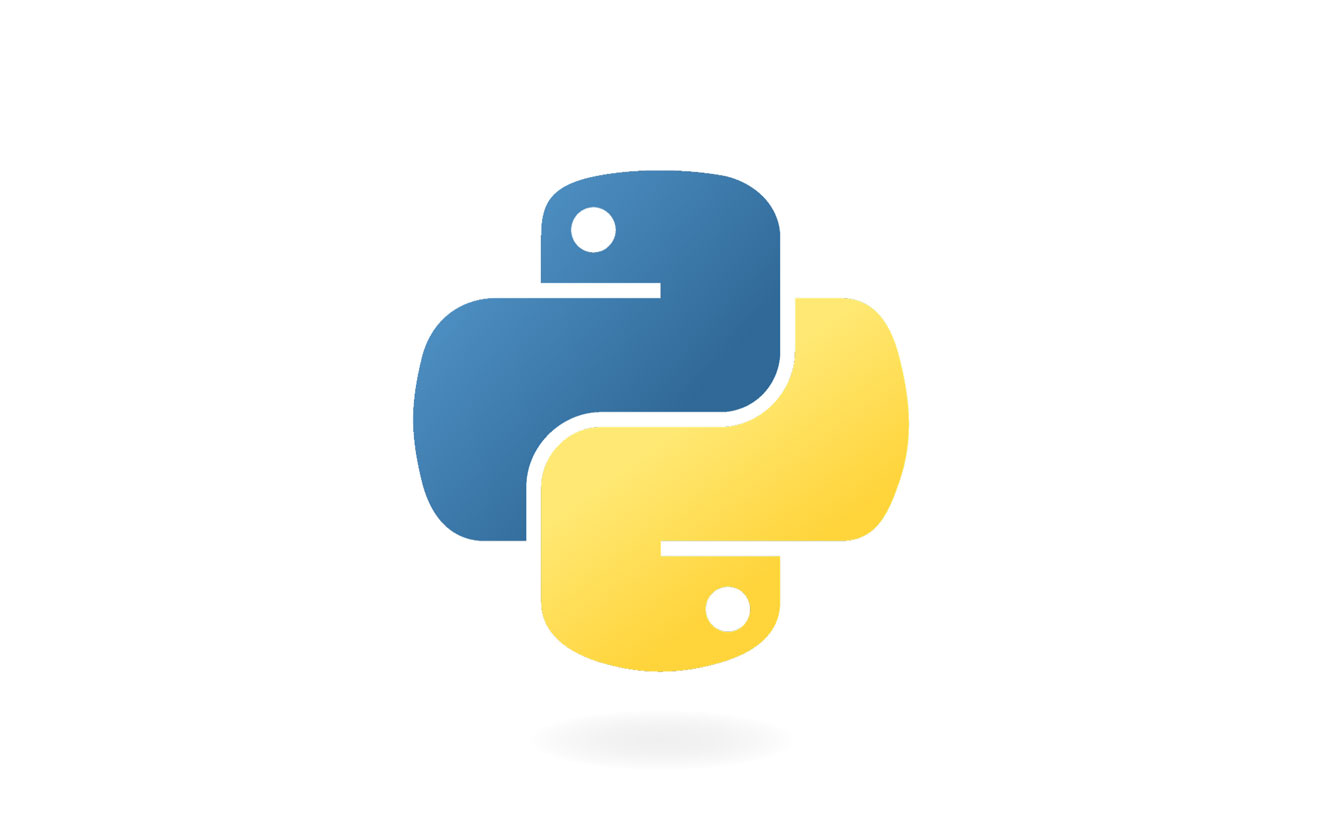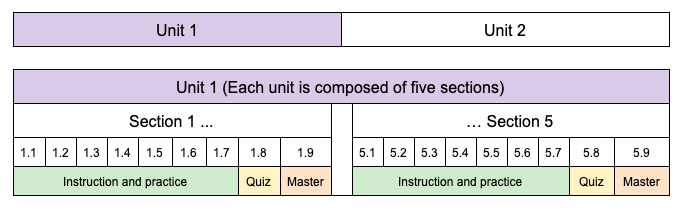In this college-level course trainees learn object-oriented programming in Python, along with selected advanced concepts including ternary operators, anonymous lambda functions, filters, maps, decorators, JSON, XML, concurrency, event-driven programming, and the basics of working with data.## Course Features

• Trainees learn at their own pace by reading tutorials, watching videos, going through examples, and solving programming challenges.
• Every short lesson is followed by self-assessment, so that trainees instantly know whether they have mastered the concept.
• Trainees obtain real-time help from the NCLab AI tutorial engine, as well as remote assistance from live course instructors as needed.
• The course incorporates fundamentals of Computer Science which every software developer should know.
• Trainees learn how to use powerful Python libraries including Matplotlib, Numpy and Scipy.
• An interactive Python coding app allows trainees to create portfolio artifacts and easily share them online.

## Prerequisites

The prerequisite for this course are Python Fundamentals.

## Equipment Requirements

Internet access

Email

One of the following browsers:

• Mozilla Firefox
• Microsoft Internet Explorer (9.0 or above)
• Safari

## Course Structure and Length

This course is self-paced, and trainees practice each skill and concept as they go.  Automatic feedback is built into the course for both practices and quizzes.

The course is divided into four Units, and each Unit is composed of five Sections. Each Section consists of 7 instructional/practice levels, a quiz, and a master (proficiency) level. Trainees can return to any level or quiz for review.While learning skills in the Advanced Python course, trainees can practice writing code, work with libraries and create portfolio artifacts with NCLab’s Python app.

Advanced Python is designed to take approximately 80-120 hours. Since the course is self-paced, the amount of time required to complete the course will vary from trainee to trainee. Trainees are responsible for learning both the tutorial content and the skills acquired through practice.

## Unit 1 (Programming Practice and Review)

While writing Python programs, trainees will also learn how binary computers work, including binary and hex numbers, logic gates, logic circuits, binary adders and multipliers. At the end of this Unit, they will have implemented a complete model of a binary computer in Python.

### Section 1

• Understand why decimal numbers are natural for humans, but binary numbers are natural for computers.
• Know that binary numbers consist of zeros and ones.
• Count in binary.
• Know the different types of variables, such as integer (int), real number (float), text string (str) or a list (list).
• Check types of variables using the built-in Boolean function isinstance().
• Generate random integers using the function randint() from the random library.
• Generate random binary numbers.
• Convert decimal numbers to binary.
• Convert binary numbers to decimal.
• Review adding positive integers using the pencil-and-paper method.
• Add binary numbers in base 2.
• Know that computers first convert decimal numbers into binary, then process them, and then convert the result back into decimal again.

### Section 2

• Know the meaning of bits (b), Bytes (B), and many other units of data size.
• One bit (1 b) represents the smallest amount of information – two objects or states such as True or False, 1 or 0, etc.
• With N bits one can represent 2**N objects. For example, with 8 bits one can represent 2**8 = 256 objects such as text characters.
• 8 b = 1 B, 1024 B = 1 KB, 1024 KB = 1 MB, 1024 MB = 1 GB, 1024 GB = 1 TB etc.
• Units such as Bytes (B), Kilobytes (KB), Megabytes (MB) etc. are mostly used for the size of stationary data.
• Know what amount of data these units can roughly represent.
• Units such as Kilobits per second (Kbps), Megabits per second (Mbps) etc. are used to indicate speed of streaming data.
• Use the ASCII table to represent text characters.
• One ASCII character is represented using 8 bits (1 Byte).
• The ASCII table contains 256 characters.
• The first 32 ASCII characters are control characters and the last 128 are other special non-printable characters.
• There are 95 printable characters that begin with the empty space (code 32).
• Obtain the ASCII code of a given text character using the function ord().
• Obtain the text character for a given ASCII code using the function chr().
• Implement and use the famous ROT13 cipher. The ROT13 cipher is special because it can be used for both encryption and decryption.
• Use the ASCII table to convert a text string into a binary stream and vice versa.
• Add (concatenate) text strings on the binary level.

### Section 3

• Work with hexadecimal (hex) numbers.
• Know that one hex digit ‘0’…’F’ corresponds to decimal 0…15.
• Count in the hexadecimal system.
• Know that two main applications of hex numbers are representing locations (addresses) in computer memory and colors.
• Check whether a given item is present in a list.
• Use the ASCII table when generating hex numbers.
• Convert decimal numbers to hexadecimal.
• Convert hexadecimal numbers to decimal.
• Uppercase hex numbers before working with them, because they may contain both lowercase or uppercase characters.
• Know the special relationship between hexadecimal and binary numbers. More precisely, that one hex digit exactly corresponds to four binary digits.
• Convert hex numbers convert to binary one digit at a time.
• Convert binary numbers to hexadecimal in four digit chunks.
• Use Exceptions to check the correctness of input data in functions.
• Sometimes these sanity checks can take more lines than the actual function body – that is perfectly OK.

### Section 4

• Learn about transistor count, Moore’s Law, and CPU clock speed.
• Explore transistors and logic gates.
• Use Python to simulate the basic seven types of logic gates AND, OR, NOT, NAND, NOR,  XOR, XNOR.
• Practice safe programming with Exceptions

### Section 5

• Use the logic gates to build the Half Adder, and understand that it represents a fundamental connection between logic and arithmetic.
• Build different adders and multipliers, including:
• Full (1-Bit) Adder using logic gates.
• Two-Bit, Four-Bit, and Eight-Bit Adders.
• Multiply binary numbers by combining the shift and sum operations.
• Four-Bit Multiplier (FBM).
• Decimal multiplier based on the FBM.

## Unit 2 (Object-Oriented Programming)

In this Unit, trainees will gain a detailed understanding of Object-Oriented Programming (OOP) in Python. At the end of this Unit, they will have implemented their own Turtle Graphics and upgraded their Graphics Editor to an OOP design.

### Section 6

• Understand the difference between procedural (imperative) and object-oriented programming.
• Explain the philosophy of OOP, including its pros and cons, and how encapsulation makes software projects easier to manage.
• Create a new class using the keywords class and object.
• Create and understand the purpose of constructors.
• The keyword self must be the first parameter of the constructor and all methods.
• All attributes and methods of a class are accessed through the prefix self.
• Add new methods and new attributes to a class.
• Instantiate a class.
• Create class Line.

### Section 7

• Use the class Line from Section 1 to implement a simplified version of the famous Python interactive Turtle Graphics drawing program.
• Create class Turtle.
• Understand that all Python methods and attributes are public; whereas C++ and Java also have private and protected methods and attributes.
• Understand that a class should always manipulate its attributes using its own methods.

### Section 8

• Derive subclasses and create class hierarchies, understand inheritance.
• Upgrade the Graphics Editor from Unit 2 to an object-oriented design (Part 1).
• Create a base class Shape.
• Teach it universal methods that will apply to all subclasses, such as how to move, scale, rotate, reverse the orientation of its boundary, and merge other shapes into itself.
• In the Shape class, the geometry will be undefined (arrays xarray and yarray will be empty).
• Design a hierarchy of geometrical objects and the corresponding classes:
• All descendants of the class Shape automatically know how to move, scale, rotate etc.
• Only individual geometries are added on the level of the descendants.

### Section 9

• Inspect classes and objects. Finalize the upgrade of the Graphics Editor to an object-oriented design.
• Inspect classes and objects.
• Check if an object is an instance of a given class or its superclass.
• Obtain the class corresponding to a given object.
• Check whether an object has a given attribute or method.
• Check whether a class is a subclass of another class.
• Obtain the class from an instance.
• Obtain the class name from a given instance.

### Section 10

• Use polymorphism to redefine the characteristics of an ancestor class from a derived class.
• Understand multiple inheritance and its drawbacks.

In this Unit, trainees will learn selected advanced techniques of Python programming.

### Section 11

• Functions with a variable number of parameters (*args and **kwargs).

### Section 12

• Lambda expressions and anonymous functions.
• When anonymous functions should and should not be used.

### Section 13

• Filters and maps, and how lambda functions are used in them.
• Function reduce().
• Conditional statements (ternary operators).

• JSON
• XML

### Section 15

• Concurrency and event-driven programming.

## Unit 4 (Working With Data)

In this Unit, trainees will learn how to use powerful Python libraries Pandas, Seaborn and Statsmodels to perform elementary operations with data.

### Section 16

• Importing the Pandas, Seaborn and Matplotlib libraries.
• Understanding Pandas DataFrames.
• Entering data into DataFrames.
• Using the regression line to make predictions.

### Section 17

• Reading files from the hard disk or from a URL.
• Obtaining the number of rows and number of columns of a DataFrame.
• Obtaining the list of all column names of a DataFrame.
• Displaying the beginning and the end of a DataFrame.

### Section 18

• Calculating the minimum, maximum, sum and mean of column values.
• Calculating variance and standard deviation.
• Plotting histograms with Pandas.
• Displaying boxplots with Seaborn, and how to interpret them.

### Section 19

• Correlation and the Pearson coefficient (R-value).
• Visualizing correlation graphically via heatmaps.
• Understanding the purpose of using the R-squared value, and its advantages over the R-value.
• Calculating the R-squared value by squaring all values in the correlation matrix.

### Section 20

• Quantifying the results of linear regression analysis.
• Statistical hypothesis testing.
• Multiple linear regression.
• Logistic regression.